<< Aqua Planet: Transients from 'control' Experiment >>

resolution: T39L48, cumulus parameterization: Kuo scheme,
Original data produced: 2004/05 on ES.

 control: [GT] [SH] [TR] [ML] [MF] [PF] [TR_etc] [TR_wk] [TR_qtuz] [TR_qtuz_etc] [COMP] peaked: [GT] [SH] [TR] [ML] [MF] [PF] [TR_etc] [TR_wk] flat: [GT] [SH] [TR] [ML] [MF] [PF] [TR_etc] [TR_wk] Qobs: [GT] [SH] [TR] [ML] [MF] [PF] [TR_etc] [TR_wk] control-5N: [GT] [SH] [TR] [ML] [MF] [PF] [TR_etc] [TR_wk] 1keq: [GT] [SH] [TR] [ML] [MF] [PF] [TR_etc] [TR_wk] [SHanm] 3keq: [GT] [SH] [TR] [ML] [MF] [PF] [TR_etc] [TR_wk] [SHanm] 3kw1: [GT] [SH] [TR] [ML] [MF] [PF] [TR_etc] [TR_wk] [SHanm] flat3keq: [GT] [SH] [TR] [ML] [MF] [PF] [TR_etc] [TR_wk] [SHanm] Qobs3keq: [GT] [SH] [TR] [ML] [MF] [PF] [TR_etc] [TR_wk] [SHanm] H1998con: [GT] [SH] [TR] [ML] [MF] [PF] [TR_etc] [TR_wk] H1998pa: [GT] [SH] [TR] [ML] [MF] [PF] [TR_etc] [TR_wk] [SHanm]

3keq,Qobs3keq,flat3keq,H1998pa comparison [SHanm]
[T39L48_eml] [T39L48_ias] [T39L48_ksc] [T39L48_kuo] [T39L48_mca] [T39L48_non]
[T39L24_eml] [T39L48_eml] [T39L96_eml] [T79L48_eml] [T159L48_eml] [T319L48_eml]
[T39L24_non] [T39L48_non] [T39L96_non] [T79L48_non] [T159L48_non] [T319L48_non]
[HOME]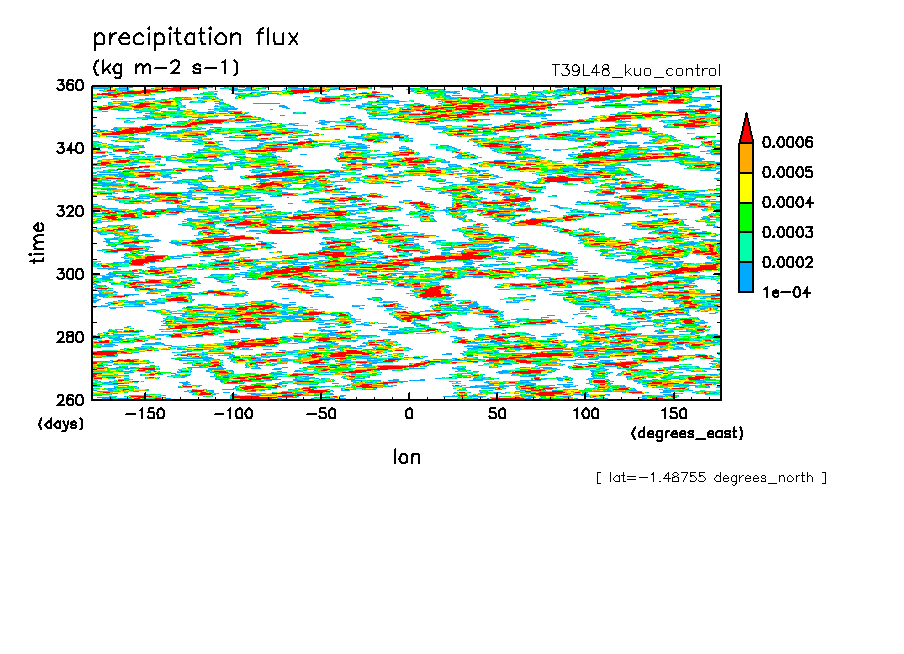tr_tppn
precipitation flux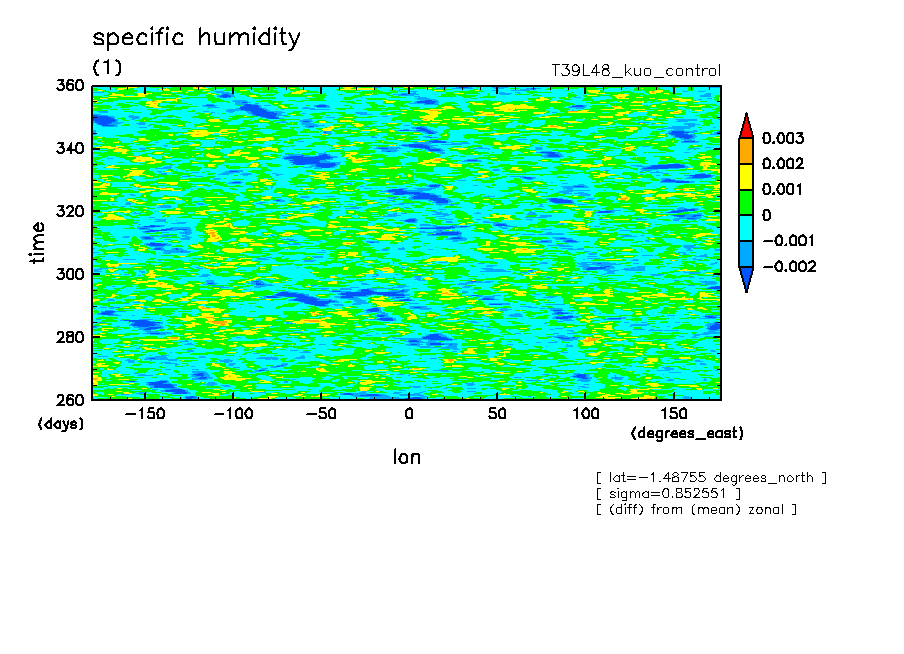tr_q850s
specific humidity (sigma=0.85)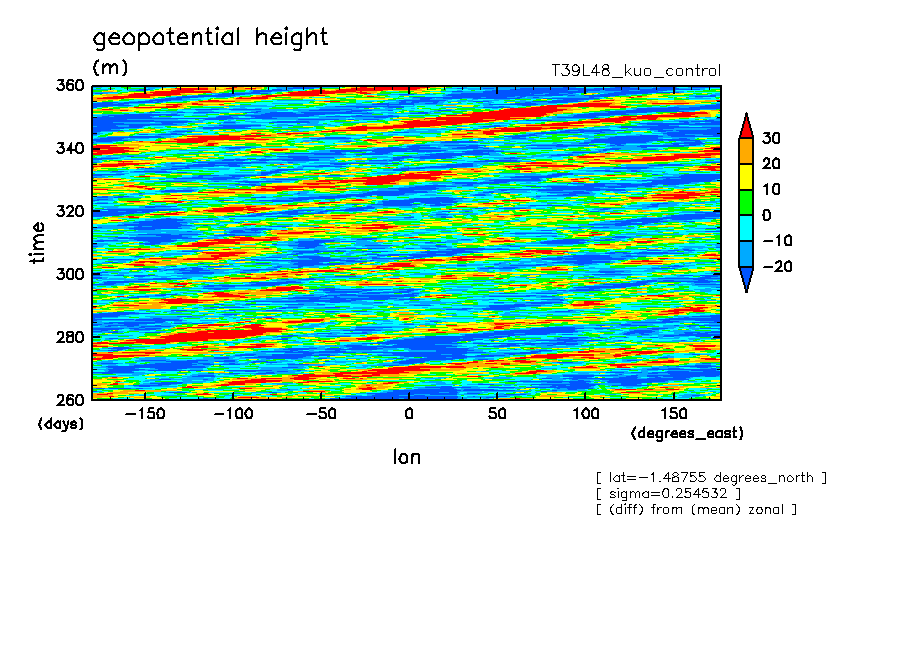tr_phi250s
geopotential height (sigma=0.25)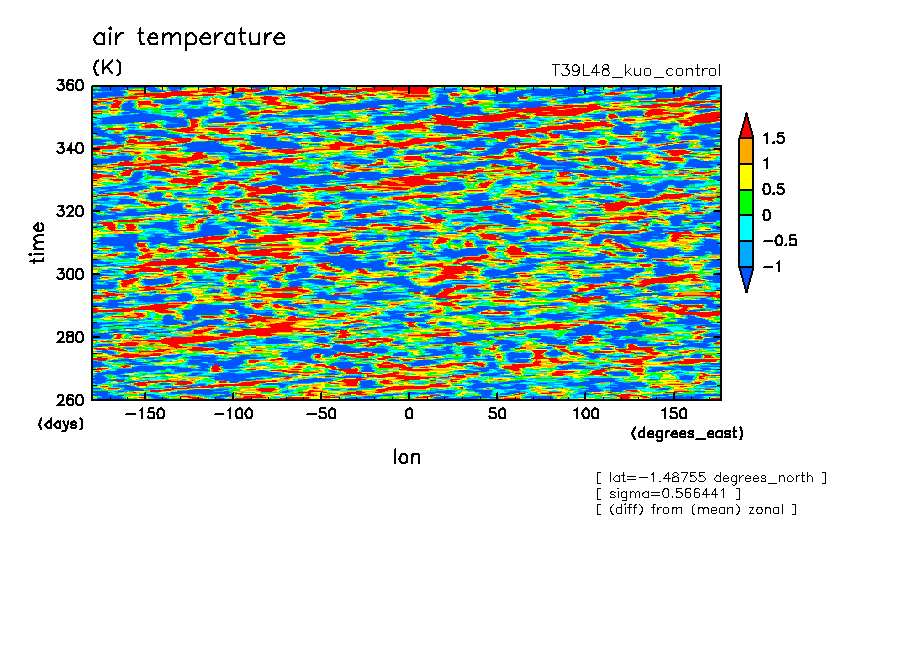tr_t570s
air temperature (sigma=0.57)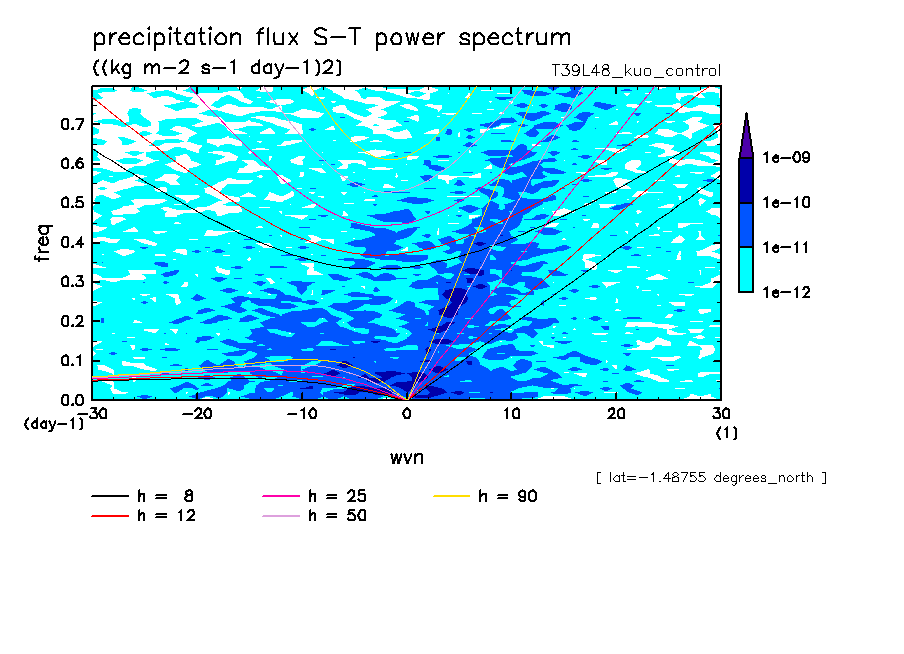tr_tppn_spct
precipitation flux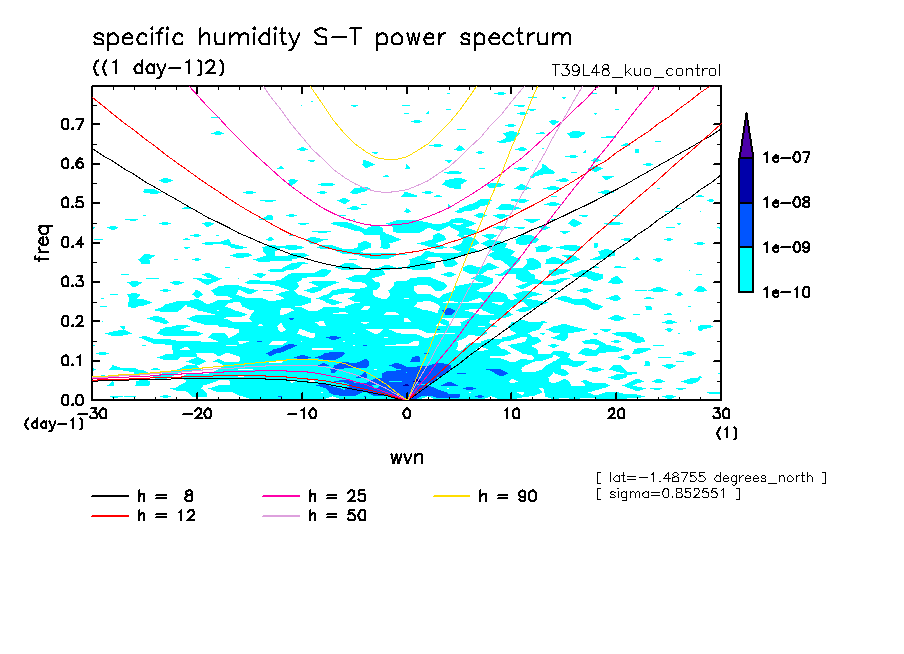tr_q850s_spct
specific humidity (sigma=0.85)tr_phi250s_spct
geopotential height (sigma=0.25)tr_t570s_spct
air temperature (sigma=0.57)tr_tppn_sym_spct
precipitation fluxtr_q850_sym_spct
specific humidity (sigma=0.85)tr_phi250_sym_spct
geopotential height (sigma=0.25)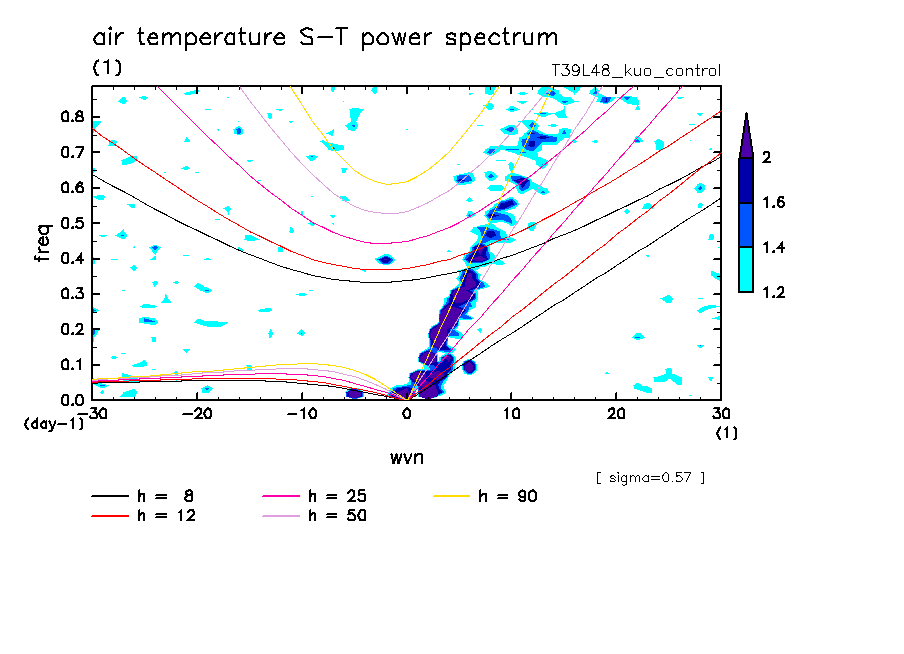tr_t570_sym_spct
air temperature (sigma=0.57)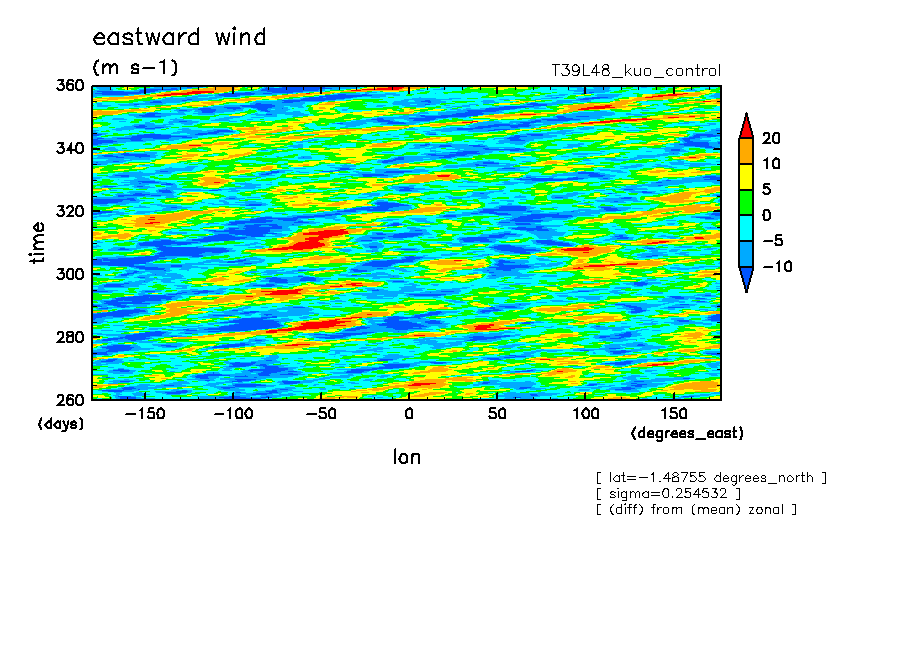tr_u250s
eastward wind (sigma=0.25)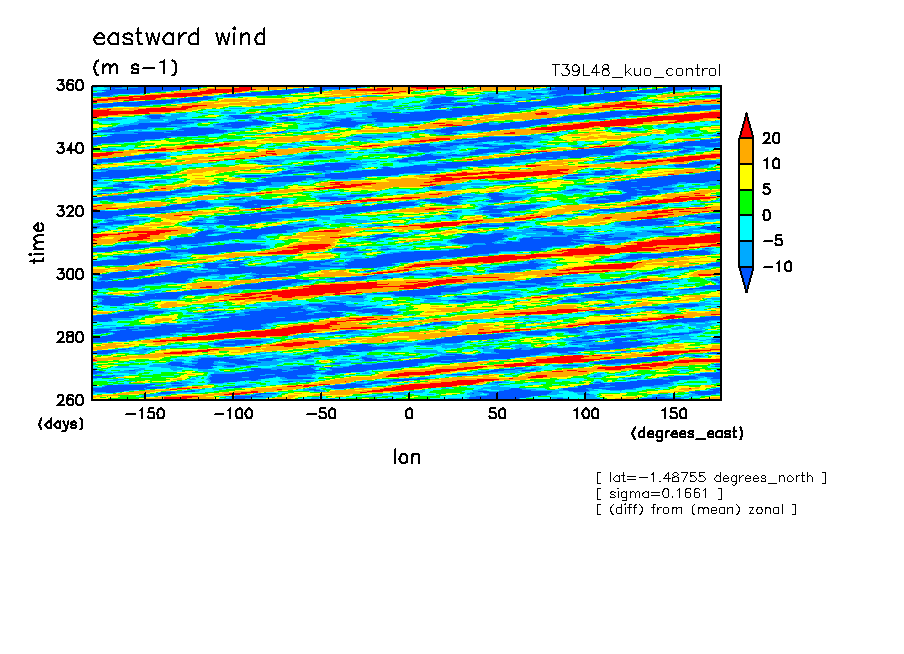tr_u170s
eastward wind (sigma=0.17)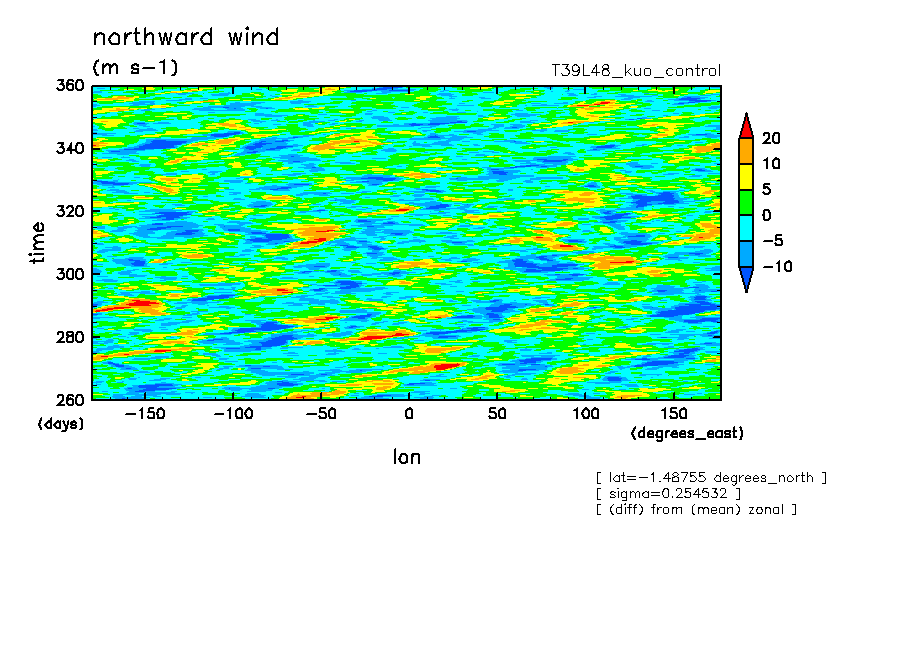tr_v250s
northward wind (sigma=0.25)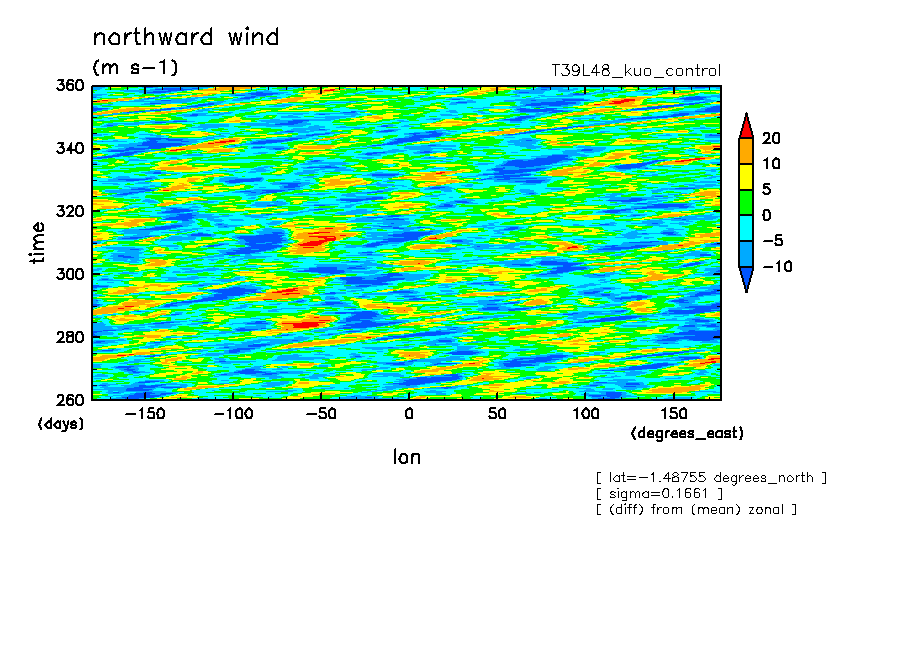tr_v170s
northward wind (sigma=0.17)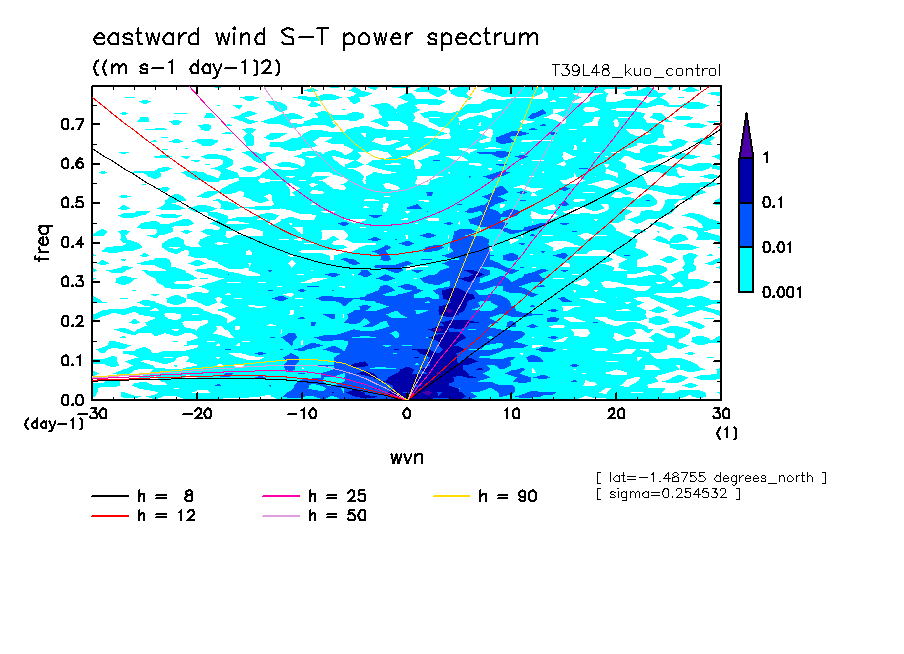tr_u250s_spct
eastward wind (sigma=0.25)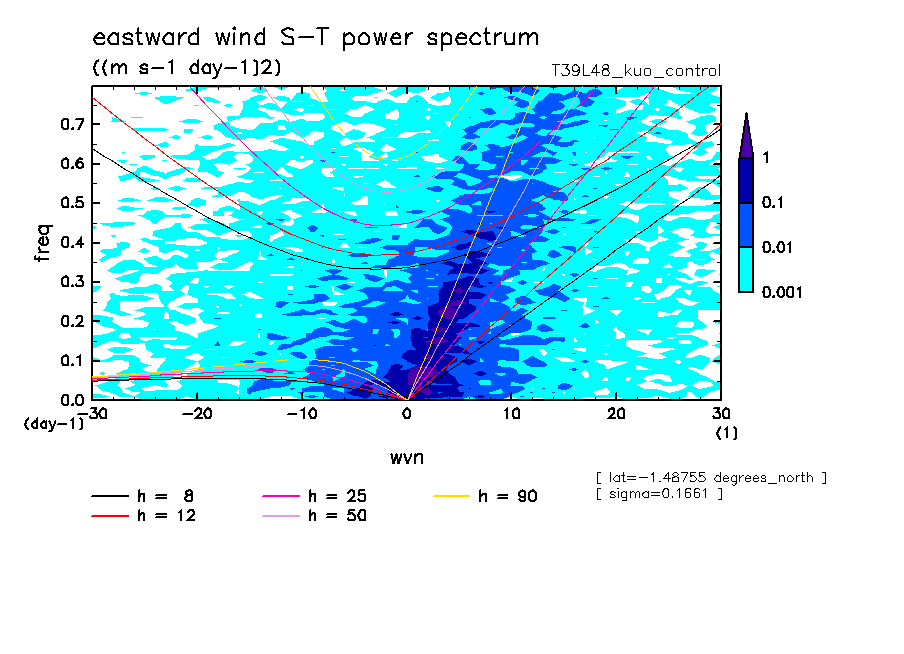tr_u170s_spct
eastward wind (sigma=0.17)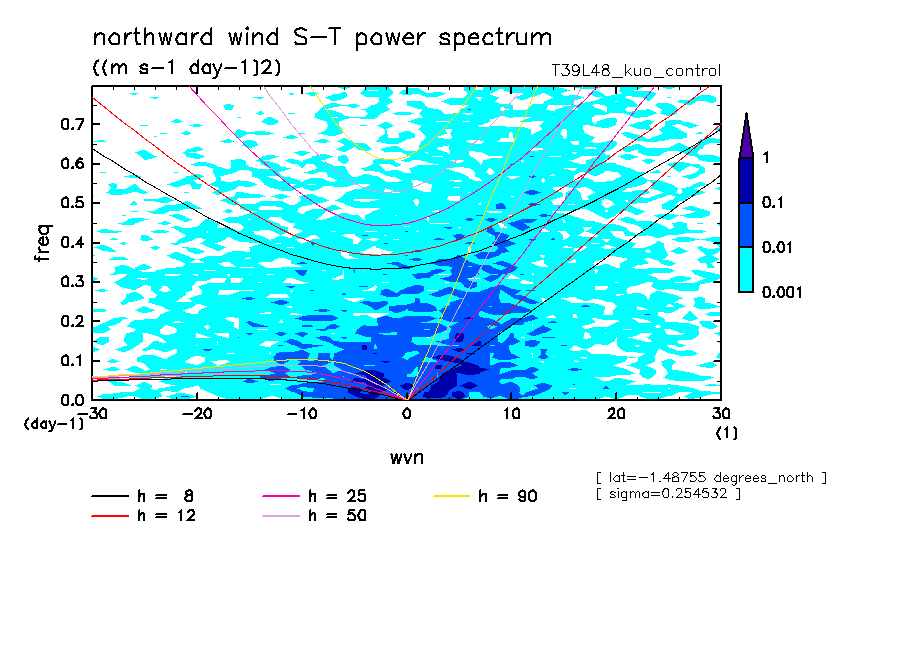tr_v250s_spct
northward wind (sigma=0.25)tr_v170s_spct
northward wind (sigma=0.17)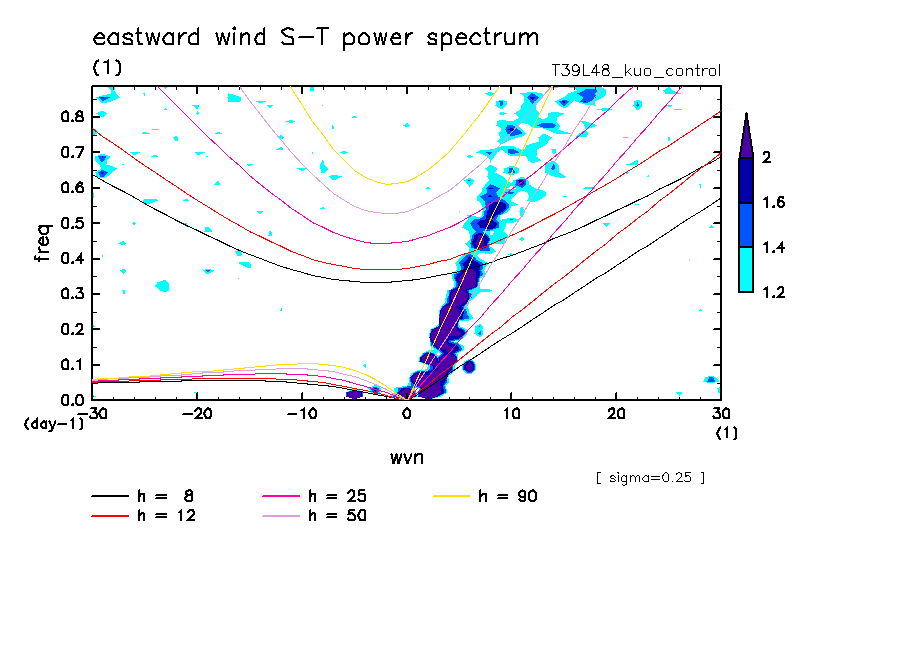tr_u250_sym_spct
eastward wind (sigma=0.25)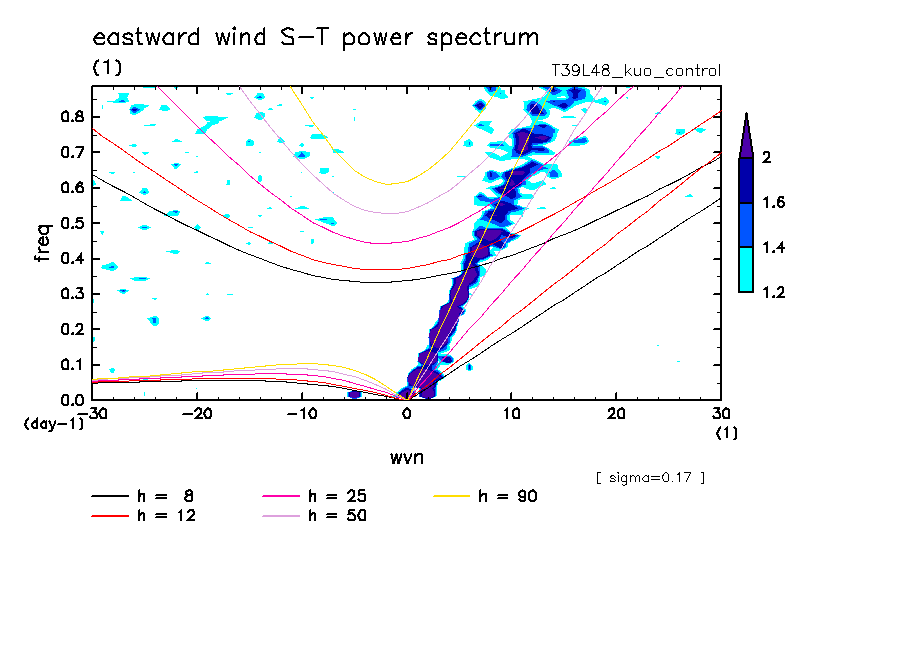tr_u170_sym_spct
eastward wind (sigma=0.17)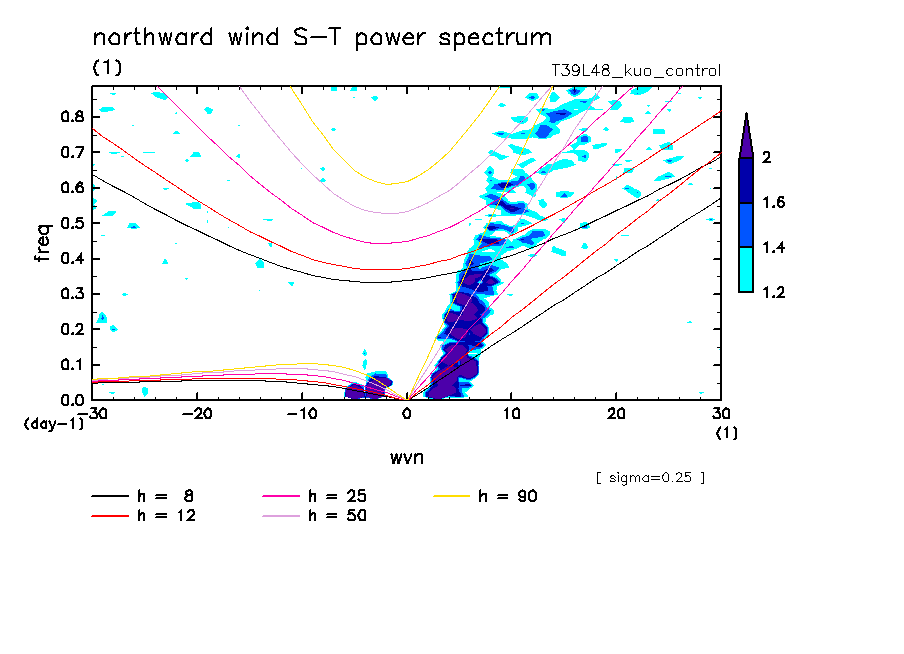tr_v250_sym_spct
northward wind (sigma=0.25)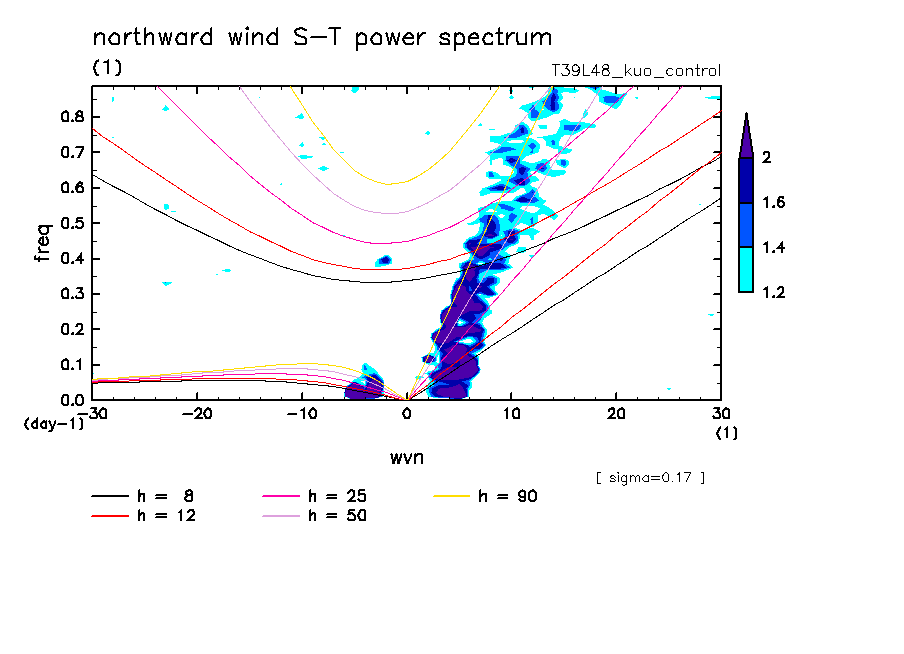tr_v170_sym_spct
northward wind (sigma=0.17)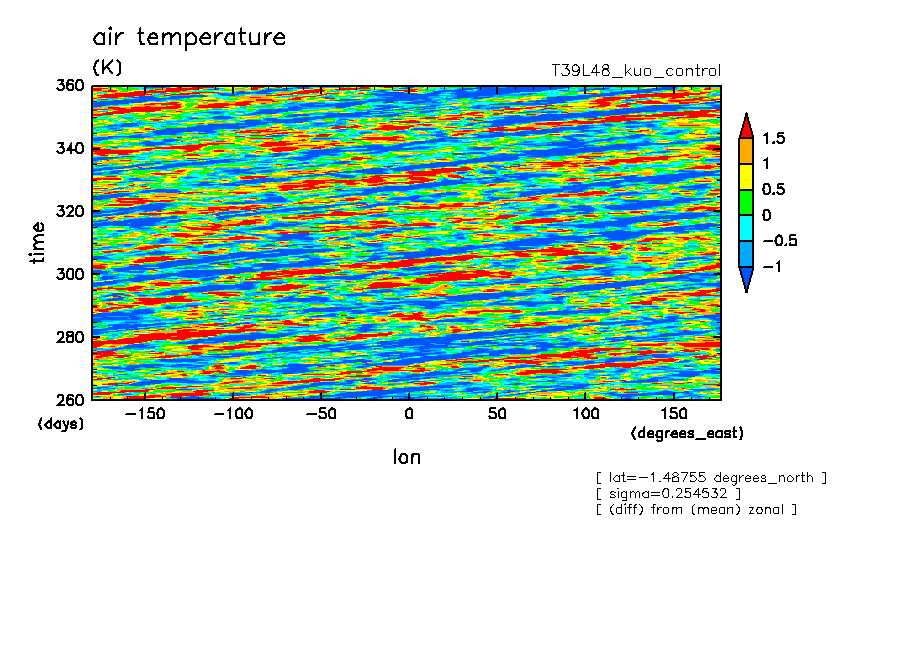tr_t250s
air temperature (sigma=0.25)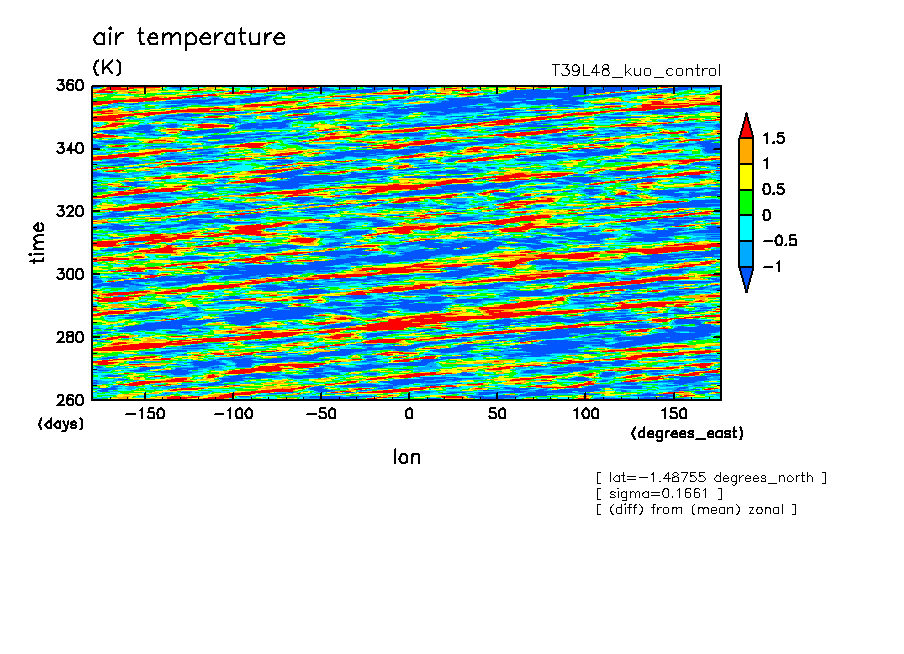tr_t170s
air temperature (sigma=0.17)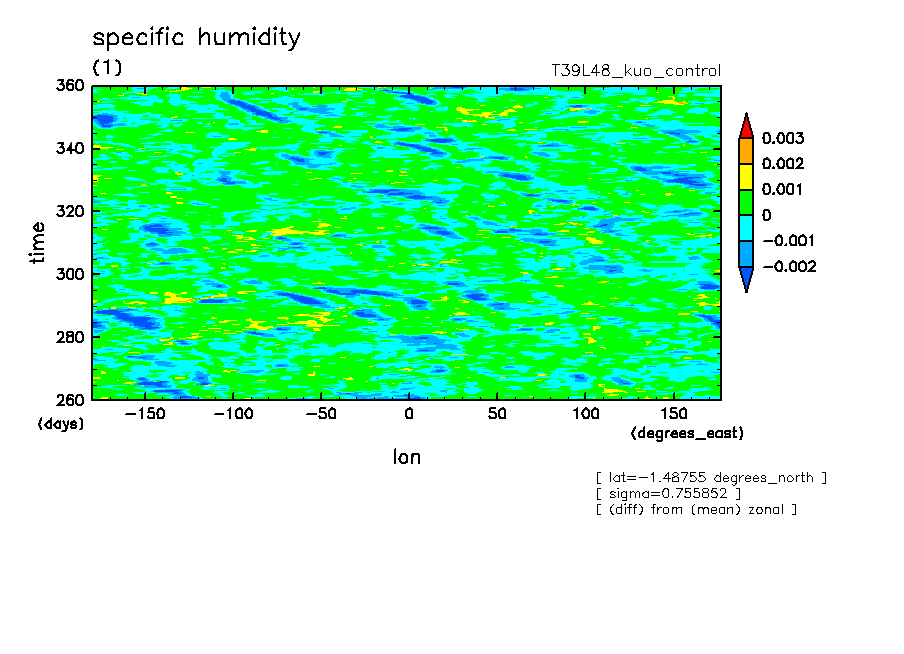tr_q760s
specific humidity (sigma=0.76)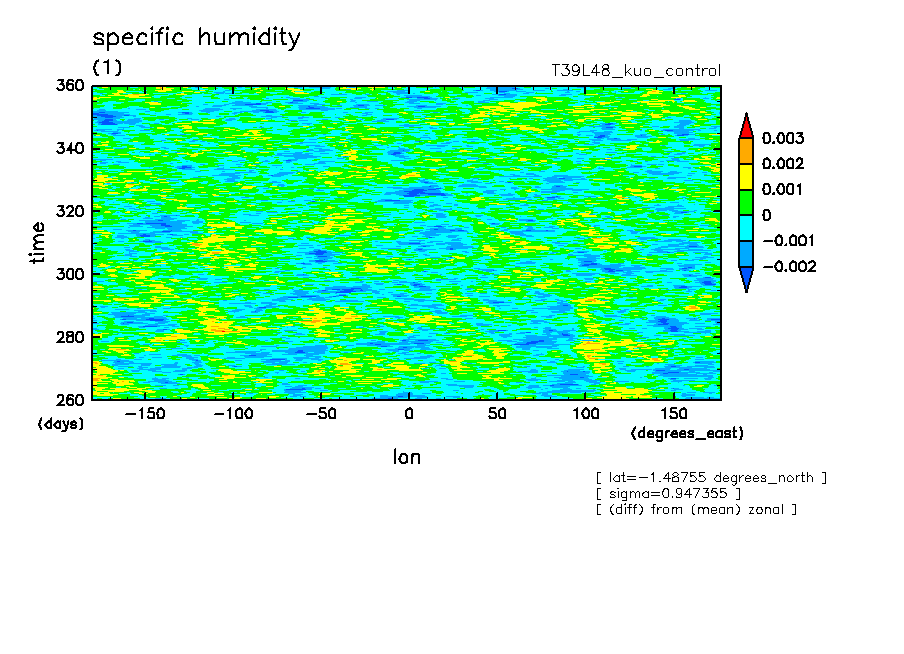tr_q950s
specific humidity (sigma=0.95)tr_t250s_spct
air temperature (sigma=0.25)tr_t170s_spct
air temperature (sigma=0.17)tr_q760s_spct
specific humidity (sigma=0.76)tr_q950s_spct
specific humidity (sigma=0.95)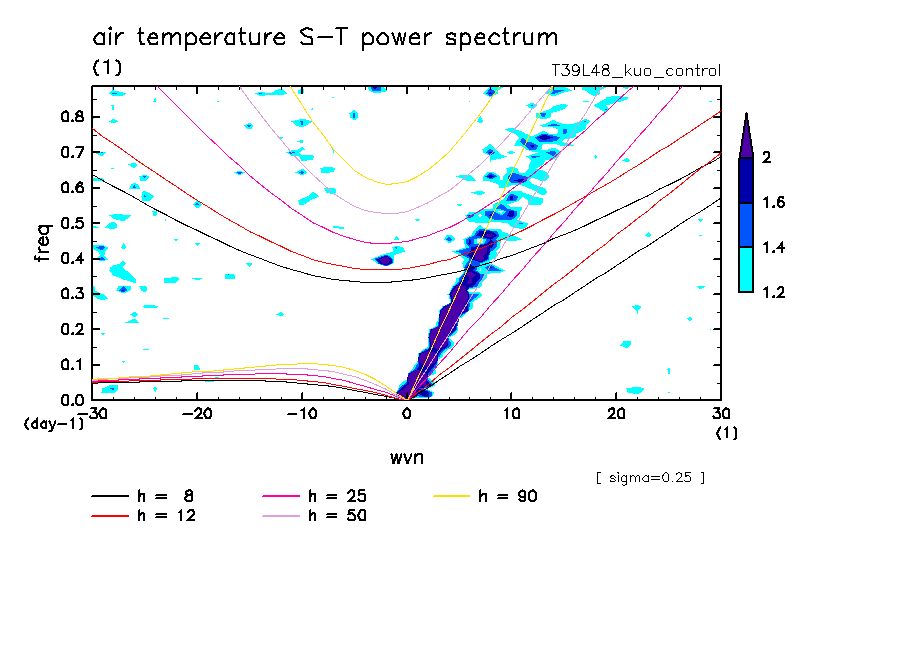tr_t250_sym_spct
air temperature (sigma=0.25)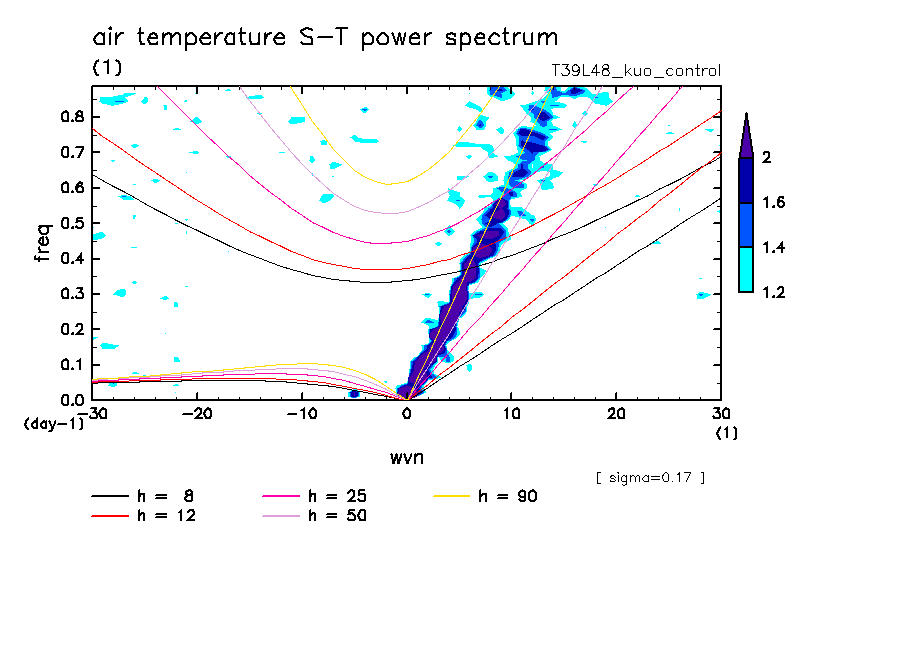tr_t170_sym_spct
air temperature (sigma=0.17)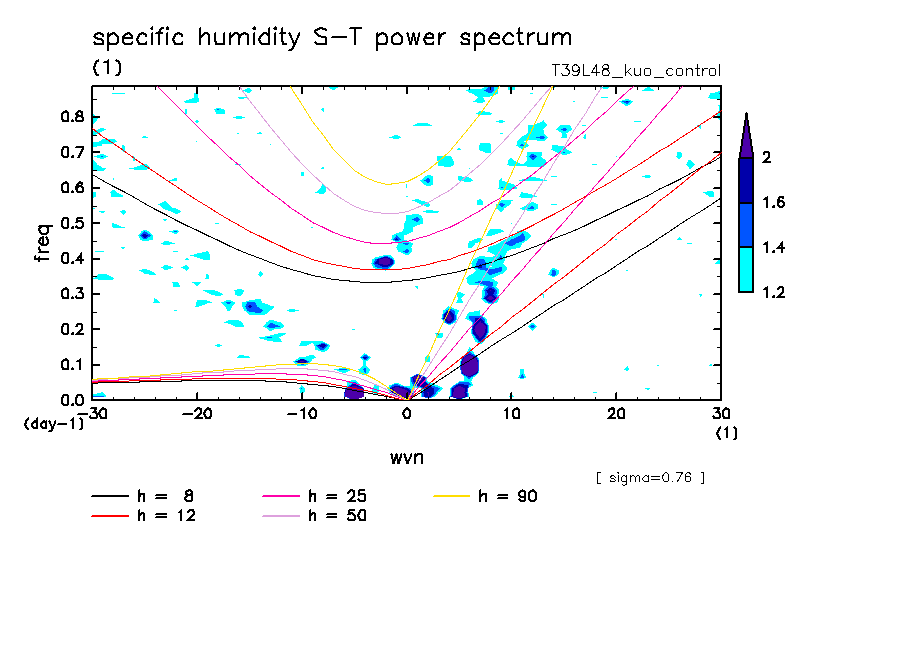tr_q760_sym_spct
specific humidity (sigma=0.76)tr_q950_sym_spct
specific humidity (sigma=0.95)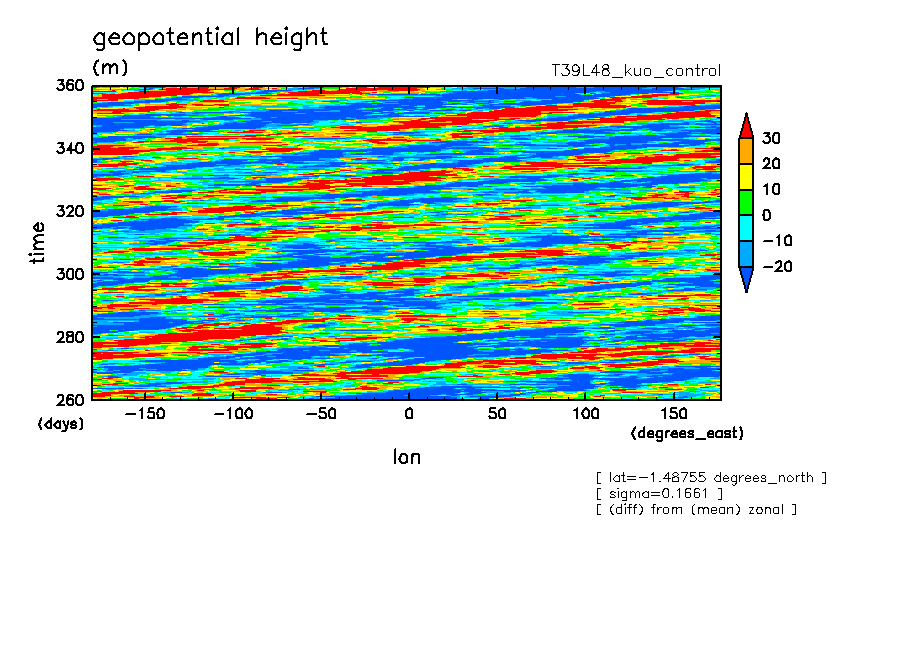tr_phi170s
geopotential height (sigma=0.17)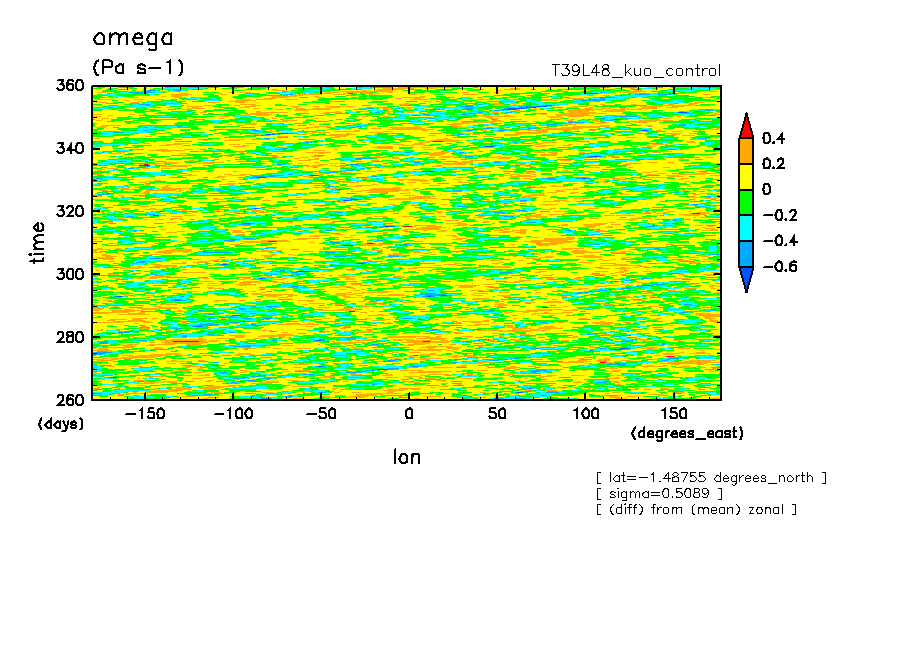tr_om500s
omega (sigma=0.50)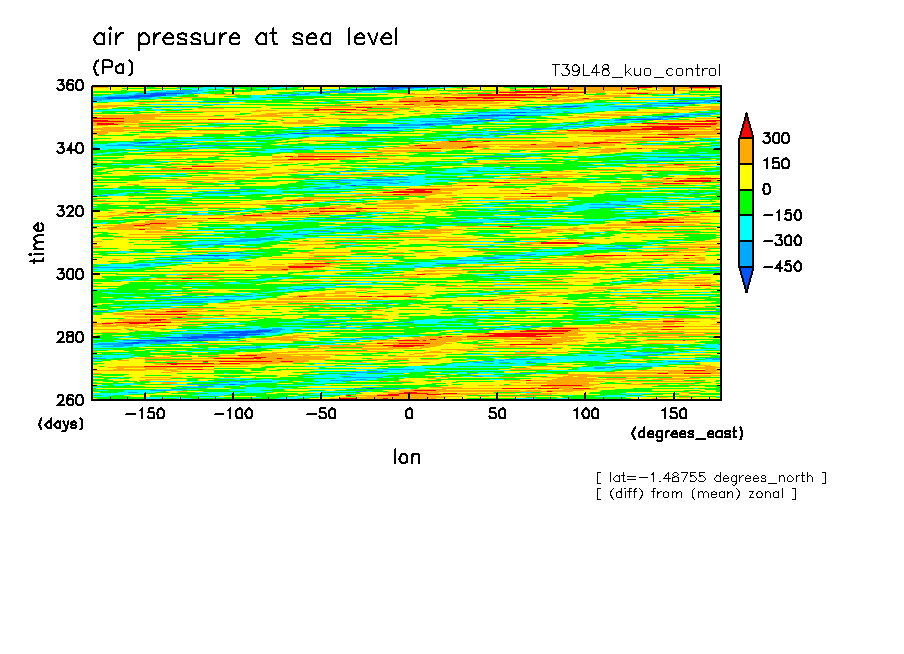tr_mslp
air pressure at sea level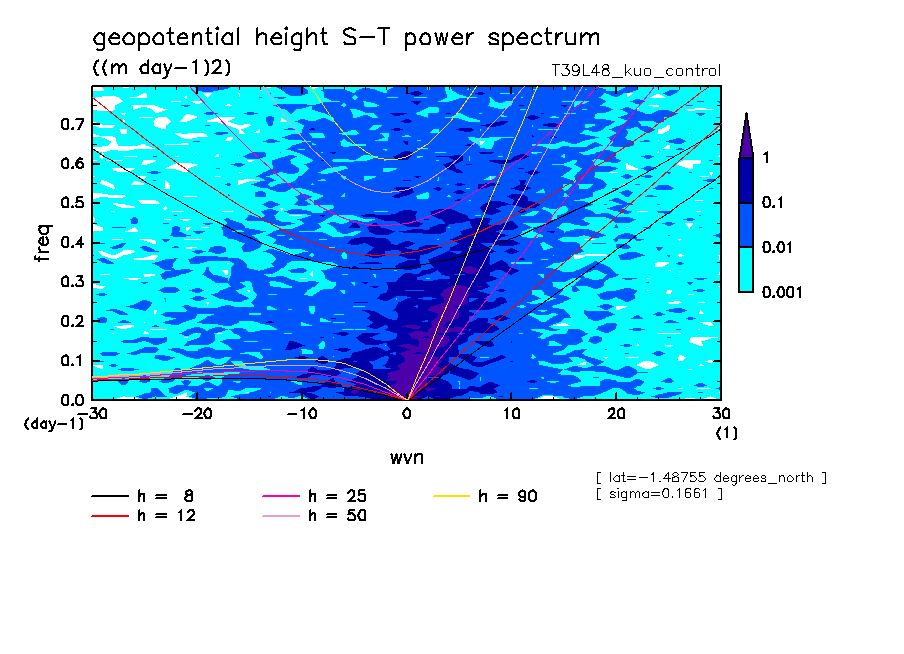tr_phi170s_spct
geopotential height (sigma=0.17)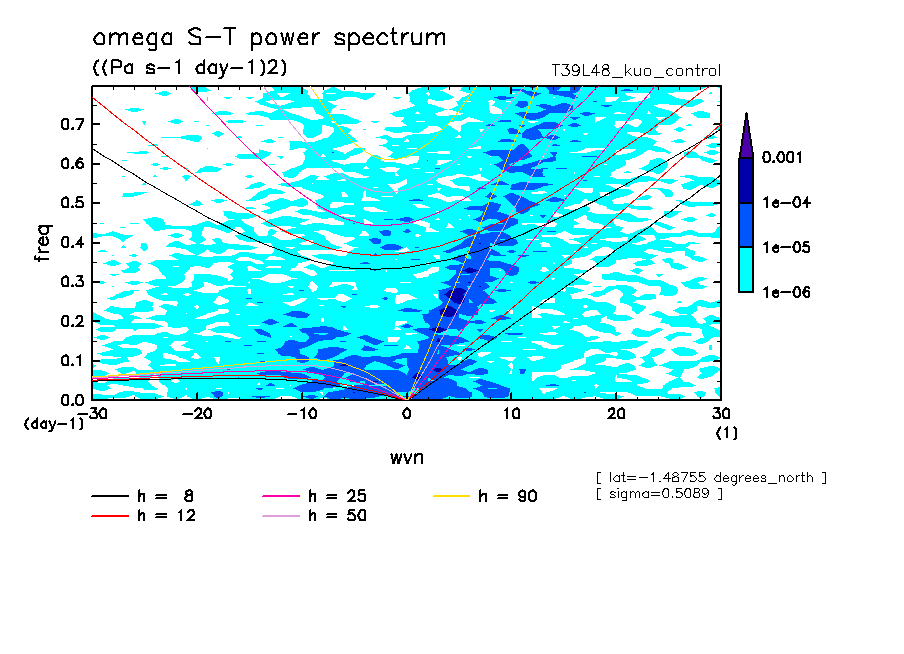tr_om500s_spct
omega (sigma=0.50)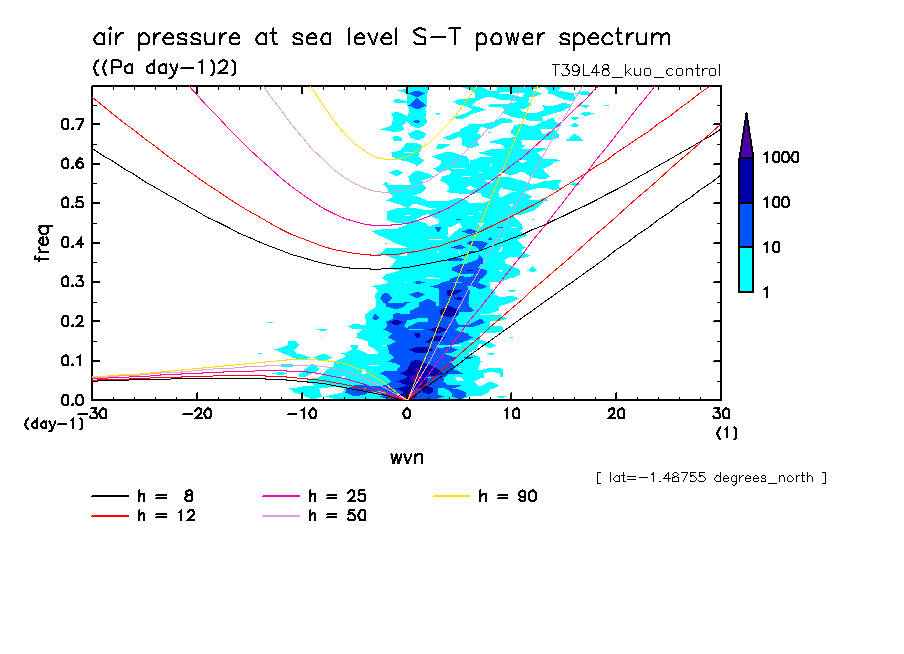tr_mslp_spct
air pressure at sea leveltr_phi170_sym_spct
geopotential height (sigma=0.17)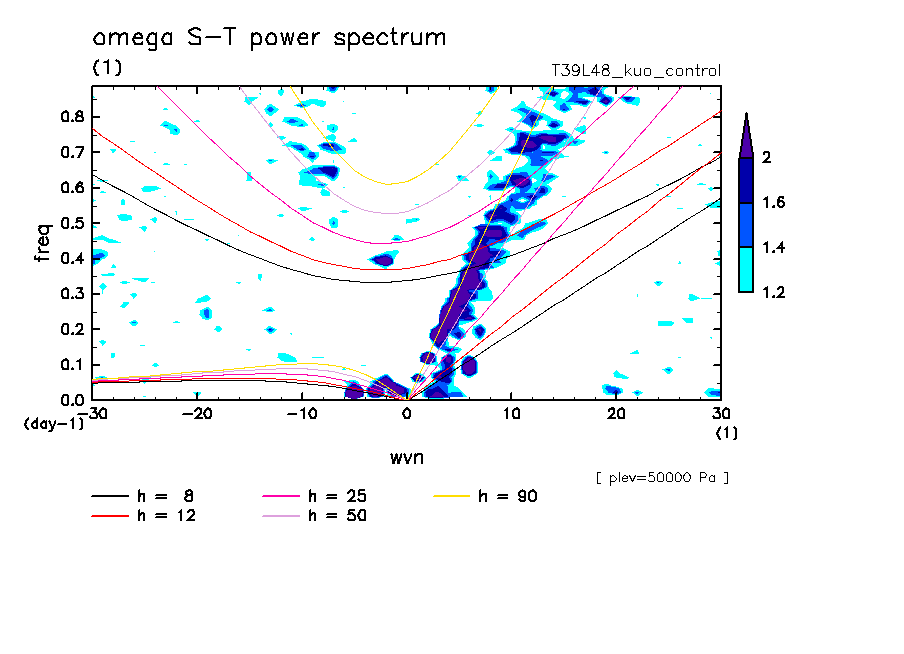tr_om500_sym_spct
omega (sigma=0.50)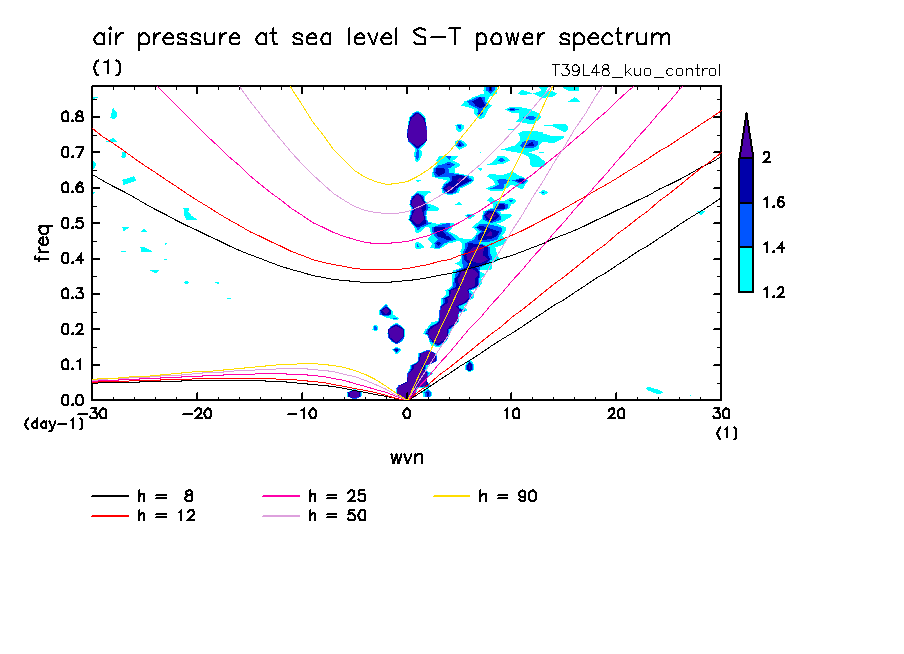tr_mslp_sym_spct
air pressure at sea level

Last update: Tue Sep 11 15:30:35 JST 2007